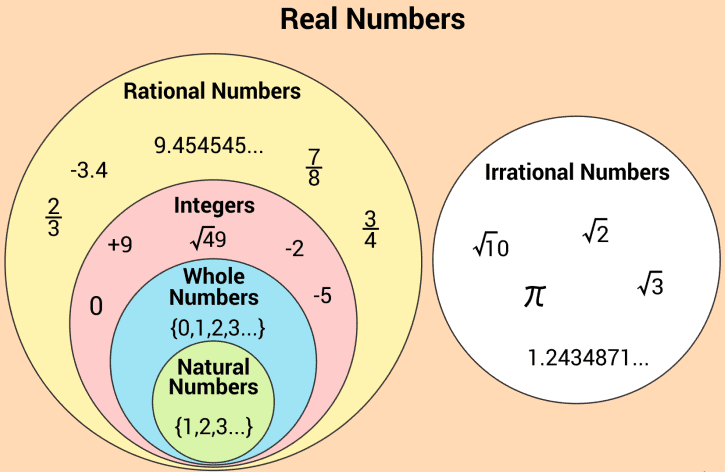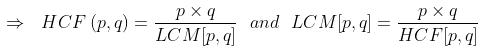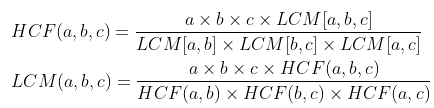Courses

# Important definitions and formulas - Real Numbers Class 10 Notes | EduRev

## Class 10 : Important definitions and formulas - Real Numbers Class 10 Notes | EduRev

The document Important definitions and formulas - Real Numbers Class 10 Notes | EduRev is a part of the Class 10 Course Mathematics (Maths) Class 10.
All you need of Class 10 at this link: Class 10

Real Numbers

• Real numbers constitute the union of all rational and irrational numbers.• In general, all the arithmetic operations can be performed on these numbers and they can be represented on the number line, also.

Euclid’s Division Lemma

• Given positive integers a and b, there exist unique integers q and r satisfying:
a = bq + r, 0 ≤ r < b.
• This lemma is essentially equivalent to:
dividend = (divisor x quotient) + remainder
where q is called the quotient and r is called the remainder.
• Lemma is a proven statement used for proving another statement.

Euclid's Division Algorithm

• This is based on Euclid's Division Lemma. It is a method used to find the H.C.F of two numbers.
• Let there be two numbers a and b where a > b, the HCF is obtained by the following method:
Step I: We apply Euclid’s Division Lemma to find two integers q and r, such that a = bq + r, 0 ≤ r < b.
Step II: If r = 0, then b is the required HCF.
Step III: If r ≠ 0, then again obtain two integers using Euclid’s Division Lemma and continue till the remainder becomes zero. The divisor when the remainder becomes zero is the required HCF.

Note: It can be extended for all integers, except zero i.e., b ≠ 0.

Example 1: Find HCF of 56 and 72.
Solution:
Apply lemma to 56 and 72.
Take a bigger number and locate ‘b’ and ‘r’. 72 = 56 × 1 + 16
Since 16 ≠ 0, consider 56 as the new dividend and 16 as the new divisor. 56 = 16 × 3 + 8
Again, 8 ≠ 0, consider 16 as the new dividend and 8 as the new divisor. 16 = 8 × 2 + 0.
Since the remainder is zero, divisor 8 is HCF.

Try yourself:The largest number that divides 70 and 125, which leaves the remainders 5 and 8, is:

Fundamental Theorem of Arithmetic

• Every composite number can be factorised as a product of primes and this factorisation is unique, apart from the order in which the prime factors occur.
• Example: 54=2×3×3×3
• Therefore, 54 is represented as a product of prime factors (One 2 and three 3s) ignoring the arrangement of the factors.

Rational Number

• The decimal expansion of every rational number is either terminating or non-terminating repeating.
• Let x be a rational number with a terminating decimal representation. Then we can express x as p/q where p and q are coprime, and the prime factorisation of q is of the form 2n 5m, where n, m are some non-negative integers.
• Let x = p/q be a rational number such that prime factorisation of q is of the form 2n 5m, where n, m are some non-negative integers. Then x has a terminating decimal expansion.
• The rational number p/q will have a non-terminating repeating (recurring) decimal representation if, in standard form, the prime factorisation of q is not of the form 2n 5m, where n, m are some non-negative integers.
Irrational Number
• A number is irrational if and only if, its decimal representation is non-terminating and non-repeating (non-recurring).
OR
• A number that cannot be expressed in the form of p/q, q ≠ 0 and p, q ∈ I, will be an irrational number. The set of irrational numbers is generally denoted by S.
Try yourself:Find out the decimal expansion of 13/125.
Prime Number
• If p is a prime and p divides a2, then p divides a, where a is a positive integer.

LCM & HCF of Two Numbers

• For any two positive integers p and q, we have:
HCF (p,q) x LCM [p, q] = p x qExample 2: There are two positive numbers such that one exceeds the other by 20 and their LCM and HCF are 504 and 4 respectively. Find the numbers.
Solution Let the numbers be 'x' and 'x + 20'.
We know that:
HCF (p,q) x LCM (p, q) = p x q
x(x + 20) = 4 x 504
x2 + 20x = 2016
x2 + (56 - 36)x - 2016 = 0
(x + 56)(x - 36) = 0
x = -56 & x = 36
x cannot be negative.
So two numbers are: x = 36 & (x + 20) = 56

LCM & HCF of Three Numbers

• For any three positive integers a, b and c, we have:Offer running on EduRev: Apply code STAYHOME200 to get INR 200 off on our premium plan EduRev Infinity!

## Mathematics (Maths) Class 10

50 videos|347 docs|138 tests

,

,

,

,

,

,

,

,

,

,

,

,

,

,

,

,

,

,

,

,

,

;